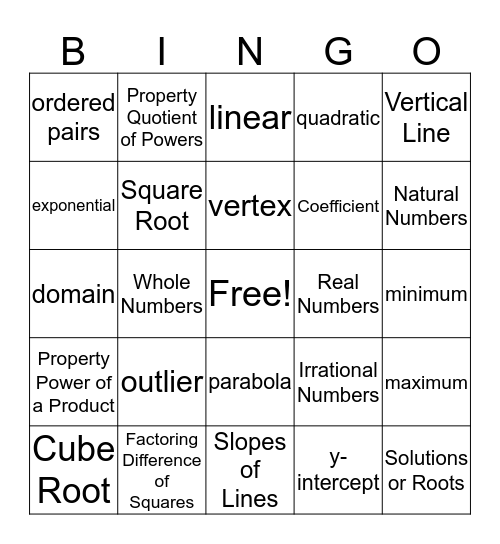# Algebra 1This bingo card has a free space and 88 words: positive, negative, parabola, radical, 4, 2, rational, irrational, integer, term, exponent, 36, maximum, minimum, zeros, vertex, axis of symmetry, substitution, slope, coefficient, constant, range, domain, distribute, y-intercept, x-intercept, function, f(x), inequality, variable, linear, quadratic, expression, equation, outlier, exponential, ordered pairs, origin, factors, Natural Numbers, Whole Numbers, Integers, Rational Numbers, Irrational Numbers, Real Numbers, Absolute Value, Order of Operations, Expression, Variable, Coefficient, Term, Exponential Form, Negative Exponent, Zero Exponent, Product of Powers, Property Power of a Power, Property Power of a Product, Property Quotient of Powers, Property Power of a Quotient, Leading Coefficient, Add Polynomials, Subtract Polynomials, Multiply Polynomials, Multiply Binomials, Factors of a Monomial, Factoring Difference of Squares, Divide Polynomials, Square Root, Cube Root, Solutions or Roots, Coordinate Plane, Linear Equation, Literal Equation, Vertical Line, Horizontal Line, Quadratic Equation, Linear Equation, Slope Formula, Slopes of Lines, Perpendicular Lines, Parallel Lines, System of Equations, Dependent variable, Independent Variable, Quadratic Formula, Relations, Function Notation and Parent Functions.

⚠ This card has duplicate items: Linear Equation (2)

## Play Online